# Star Wars Survey

Sara Stoudt true
05-14-2018

### How do perceptions of female Star Wars characters differ across age and gender?

``````require(data.table)
require(dplyr)
require(ggplot2)
setwd("~/Desktop/tidytuesday/data/2018/2018-05-14")
## read.csv didn't work for me
``````

Brute force manipulation.

``````realHeader <- sw[1, ]
sw <- sw[-1, ]

names(sw)[c(36, 38)] <- c("householdIncome", "location")
names(sw) <- "seenStarWars"
``````

Let’s focus on those who have actually seen Star Wars.

``````swYes <- subset(sw, seenStarWars == "Yes")
``````

``````## complete data only
toPlot <- swYes[-which(swYes\$Gender == ""), c("V28", "Gender", "Age")]
toPlot <- toPlot[-which(toPlot\$V28 == ""), ]

toPlot\$V28 <- factor(toPlot\$V28)
toPlot\$V28 <- factor(toPlot\$V28, levels = levels(toPlot\$V28)[c(4, 6, 3, 1, 2, 5)]) ## GROSS!

byCatGen <- toPlot %>%
group_by(V28, Gender) %>%
summarise(count = n())

byGen <- toPlot %>%
group_by(Gender) %>%
summarise(count = n())

toPlot <- byCatGen %>%
inner_join(byGen, by = c("Gender" = "Gender")) %>%
mutate(percent = count.x / count.y)
``````
``````ggplot(toPlot, aes(V28, y = percent, fill = Gender)) +
geom_bar(stat = "identity", position = position_dodge2(preserve = "total")) +
theme(axis.text.x = element_text(angle = 45, hjust = 1)) +
xlab("") +
``````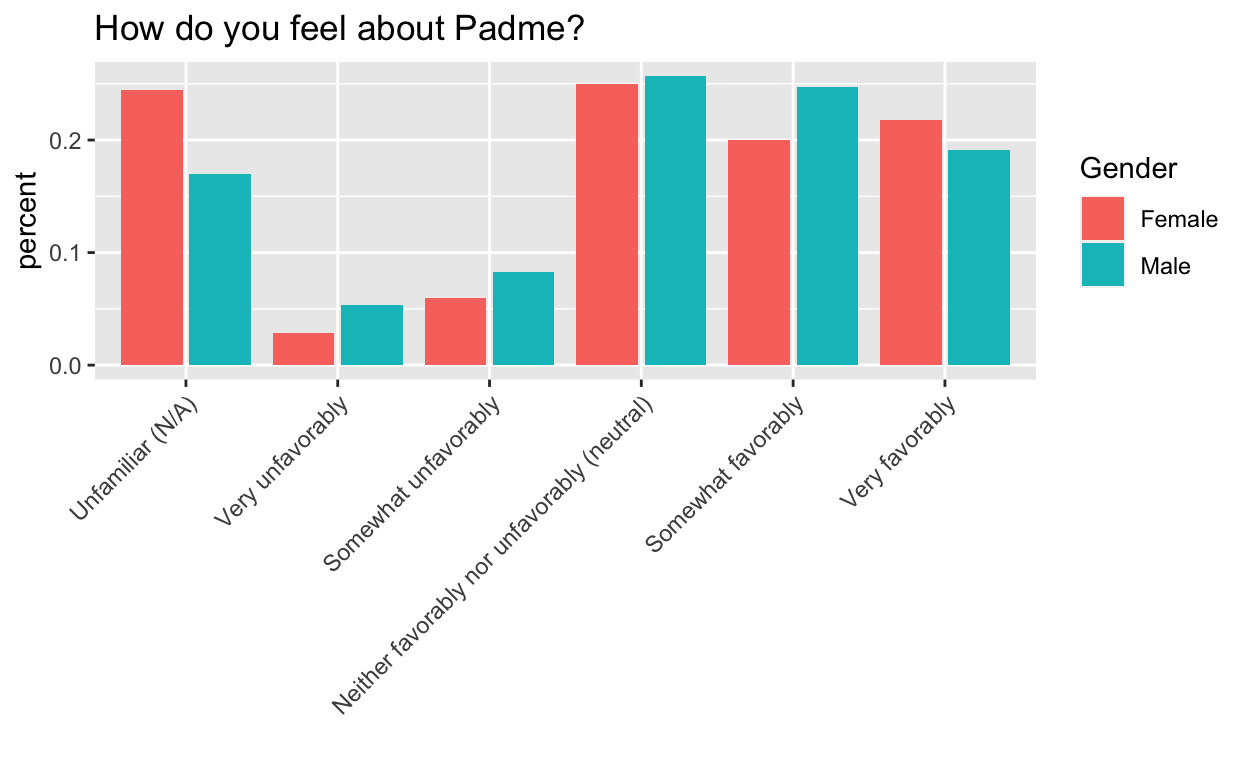``````## complete data only
toPlot <- swYes[, c("V28", "Gender", "Age")]
toPlot <- swYes[-which(swYes\$Gender == ""), c("V28", "Gender", "Age")]
toPlot <- toPlot[-which(toPlot\$V28 == ""), ]

## relevel
toPlot\$V28 <- factor(toPlot\$V28)
levels(toPlot\$V28)
``````
`````` "Neither favorably nor unfavorably (neutral)"
 "Somewhat favorably"
 "Somewhat unfavorably"
 "Unfamiliar (N/A)"
 "Very favorably"
 "Very unfavorably"                           ``````
``````toPlot\$V28 <- factor(toPlot\$V28, levels = levels(toPlot\$V28)[c(4, 6, 3, 1, 2, 5)]) ## GROSS!

byCatGenAge <- toPlot %>%
group_by(Gender, Age, V28) %>%
summarise(count = n())

byGenAge <- toPlot %>%
group_by(Gender, Age) %>%
summarise(count = n())

toPlot <- byCatGenAge %>%
inner_join(byGenAge, by = c("Gender" = "Gender", "Age" = "Age")) %>%
mutate(percent = count.x / count.y)

## get combo
toPlot\$genderAge <- paste(toPlot\$Gender, toPlot\$Age) ## is there a less hacky way to do this?
toPlot\$genderAge <- as.factor(toPlot\$genderAge)

## relevel
levels(toPlot\$genderAge)
``````
`````` "Female > 60"  "Female 18-29" "Female 30-44" "Female 45-60"
 "Male > 60"    "Male 18-29"   "Male 30-44"   "Male 45-60"  ``````
``````toPlot\$genderAge <- factor(toPlot\$genderAge, levels = levels(toPlot\$genderAge)[c(2, 6, 3, 7, 4, 8, 1, 5)]) ## GROSS!
``````

Thanks for the help!!

From @ibddoctor: https://t.co/193sOToMJB

``````levels(toPlot\$genderAge)
``````
`````` "Female 18-29" "Male 18-29"   "Female 30-44" "Male 30-44"
 "Female 45-60" "Male 45-60"   "Female > 60"  "Male > 60"   ``````
``````ggplot(toPlot, aes(V28, y = percent, fill = genderAge, order = genderAge)) +
geom_bar(stat = "identity", position = position_dodge2(preserve = "total")) +
theme(axis.text.x = element_text(angle = 45, hjust = 1)) +
xlab("") +
scale_fill_manual("legend", values = c("Female 18-29" = "indianred", "Male 18-29" = "red", "Female 30-44" = "dodgerblue", "Male 30-44" = "blue", "Female 45-60" = "green", "Male 45-60" = "forestgreen", "Female > 60" = "grey", "Male > 60" = "black"))
``````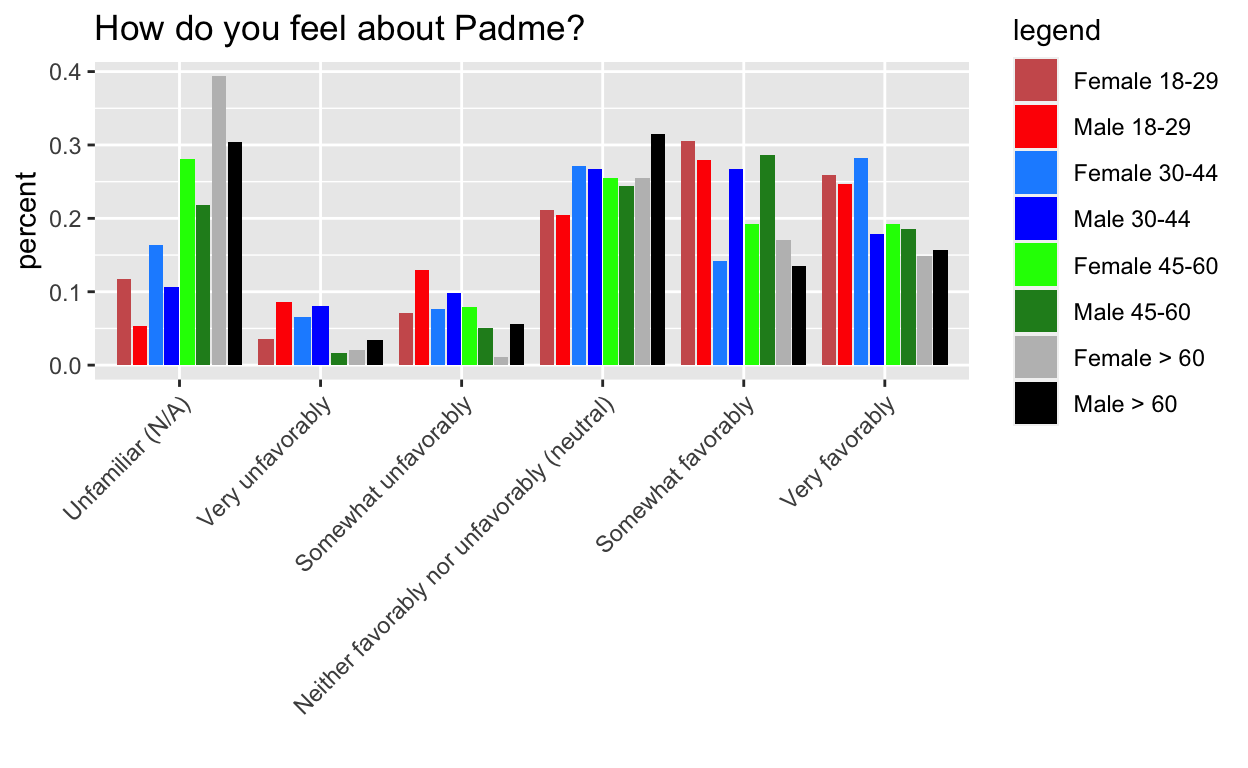``````## NOPE, but this would be ideal
``````

``````toPlot\$Gender <- as.factor(toPlot\$Gender)
toPlot\$Age <- as.factor(toPlot\$Age)

levels(toPlot\$genderAge) ## fine
``````
`````` "Female 18-29" "Male 18-29"   "Female 30-44" "Male 30-44"
 "Female 45-60" "Male 45-60"   "Female > 60"  "Male > 60"   ``````
``````levels(toPlot\$Age) ## need to relevel
``````
`` "> 60"  "18-29" "30-44" "45-60"``
``````toPlot\$Age <- factor(toPlot\$Age, levels = levels(toPlot\$Age)[c(2, 3, 4, 1)]) ## GROSS!

# toPlot\$genderAge=factor(toPlot\$genderAge,levels=levels(toPlot\$genderAge)[c(2,6,3,7,4,8,1,5)]) ## GROSS!

## if it plots one level of V28 at a time, this would make sense
test <- toPlot %>% arrange(Age, V28)
``````
``````# A tibble: 6 x 7
# Groups:   Gender, Age 
Gender Age   V28                 count.x count.y percent genderAge
<fct>  <fct> <fct>                 <int>   <int>   <dbl> <fct>
1 Female 18-29 Unfamiliar (N/A)         10      85  0.118  Female 18-…
2 Male   18-29 Unfamiliar (N/A)          5      93  0.0538 Male 18-29
3 Female 18-29 Very unfavorably          3      85  0.0353 Female 18-…
4 Male   18-29 Very unfavorably          8      93  0.0860 Male 18-29
5 Female 18-29 Somewhat unfavorab…       6      85  0.0706 Female 18-…
6 Male   18-29 Somewhat unfavorab…      12      93  0.129  Male 18-29 ``````
``````ggplot(test, aes(V28, y = percent, fill = genderAge)) +
geom_bar(stat = "identity", position = position_dodge2(preserve = "total")) +
theme(axis.text.x = element_text(angle = 45, hjust = 1)) +
xlab("") +
scale_fill_manual("legend", values = c("Female 18-29" = "indianred", "Male 18-29" = "red", "Female 30-44" = "dodgerblue", "Male 30-44" = "blue", "Female 45-60" = "green", "Male 45-60" = "forestgreen", "Female > 60" = "grey", "Male > 60" = "black"))
````````````## NOPE but closer, now all the females are together
``````

THIS IS THE ONE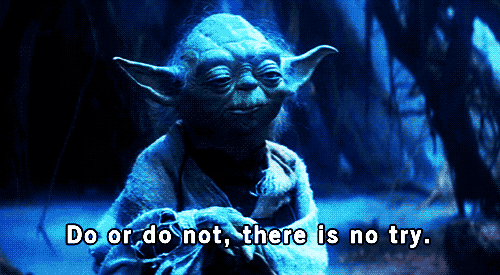Members of the older female generation are not (proportionally) Padme fans.

``````test <- as.data.frame(toPlot %>% arrange(Age, V28))
``````
``````  Gender   Age                  V28 count.x count.y    percent
1 Female 18-29     Unfamiliar (N/A)      10      85 0.11764706
2   Male 18-29     Unfamiliar (N/A)       5      93 0.05376344
3 Female 18-29     Very unfavorably       3      85 0.03529412
4   Male 18-29     Very unfavorably       8      93 0.08602151
5 Female 18-29 Somewhat unfavorably       6      85 0.07058824
6   Male 18-29 Somewhat unfavorably      12      93 0.12903226
genderAge
1 Female 18-29
2   Male 18-29
3 Female 18-29
4   Male 18-29
5 Female 18-29
6   Male 18-29``````
``````ggplot(test, aes(V28, y = percent, fill = genderAge)) +
geom_bar(stat = "identity", position = position_dodge2(preserve = "total")) +
theme(axis.text.x = element_text(angle = 45, hjust = 1)) +
xlab("") +
scale_fill_manual("legend", values = c("Female 18-29" = "indianred", "Male 18-29" = "red", "Female 30-44" = "dodgerblue", "Male 30-44" = "blue", "Female 45-60" = "green", "Male 45-60" = "forestgreen", "Female > 60" = "grey", "Male > 60" = "black"))
````````````## MAGICALLY WORKS
``````

Mystery: Why is `as.data.frame` needed?

#### Leia

Everyone loves Leia.

``````## complete data only
toPlot <- swYes[-which(swYes\$Gender == ""), c("V18", "Gender", "Age")]
toPlot <- toPlot[-which(toPlot\$V18 == ""), ]

## relevel
toPlot\$V18 <- factor(toPlot\$V18)
toPlot\$V18 <- factor(toPlot\$V18, levels = levels(toPlot\$V18)[c(4, 6, 3, 1, 2, 5)]) ## GROSS!

byCatGen <- toPlot %>%
group_by(V18, Gender) %>%
summarise(count = n())

byGen <- toPlot %>%
group_by(Gender) %>%
summarise(count = n())

toPlot <- byCatGen %>%
inner_join(byGen, by = c("Gender" = "Gender")) %>%
mutate(percent = count.x / count.y)
``````
``````ggplot(toPlot, aes(V18, y = percent, fill = Gender)) +
geom_bar(stat = "identity", position = position_dodge2(preserve = "total")) +
theme(axis.text.x = element_text(angle = 45, hjust = 1)) +
xlab("") +
ggtitle("How do you feel about Leia?")
``````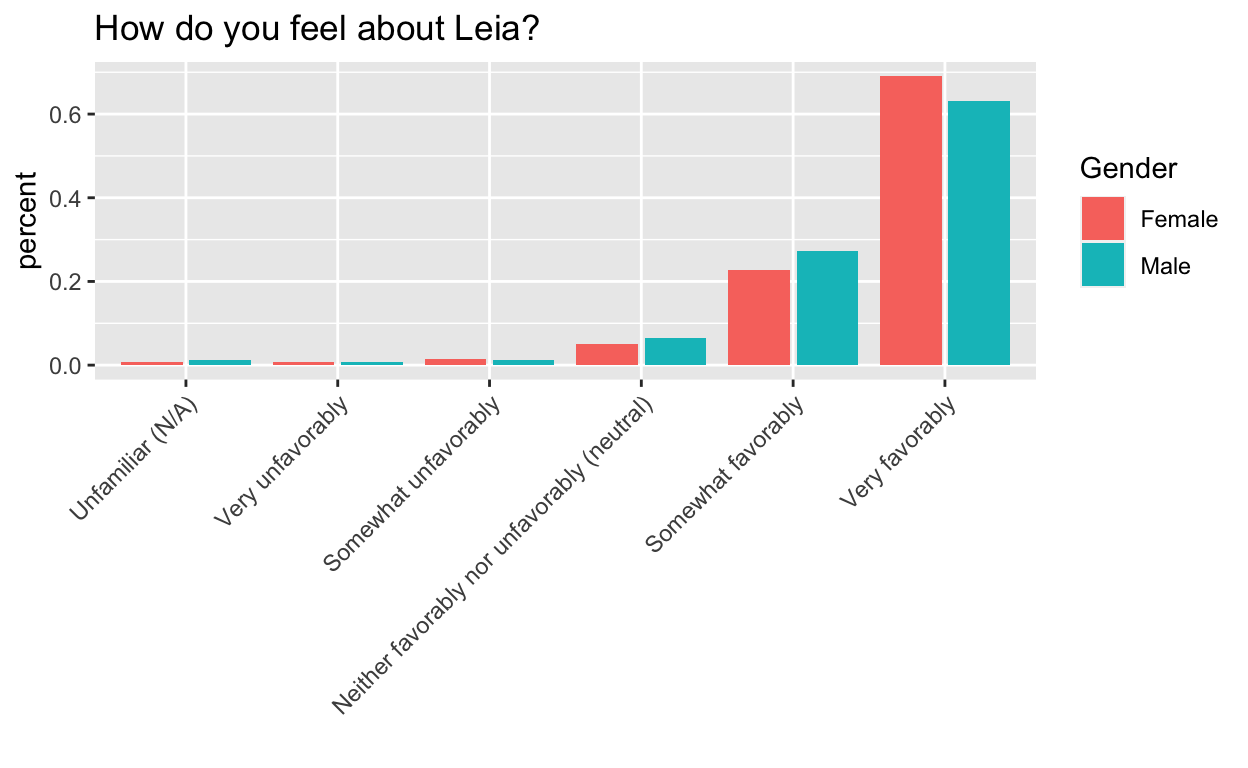``````## complete data only
toPlot <- swYes[, c("V18", "Gender", "Age")]
toPlot <- swYes[-which(swYes\$Gender == ""), c("V18", "Gender", "Age")]
toPlot <- toPlot[-which(toPlot\$V18 == ""), ]

byCatGenAge <- toPlot %>%
group_by(Gender, Age, V18) %>%
summarise(count = n())

byGenAge <- toPlot %>%
group_by(Gender, Age) %>%
summarise(count = n())

toPlot <- byCatGenAge %>%
inner_join(byGenAge, by = c("Gender" = "Gender", "Age" = "Age")) %>%
mutate(percent = count.x / count.y)

## get combo
toPlot\$genderAge <- paste(toPlot\$Gender, toPlot\$Age) ## is there a less hacky way to do this?
toPlot\$genderAge <- as.factor(toPlot\$genderAge)

## relevel
levels(toPlot\$genderAge)
``````
`````` "Female > 60"  "Female 18-29" "Female 30-44" "Female 45-60"
 "Male > 60"    "Male 18-29"   "Male 30-44"   "Male 45-60"  ``````
``````toPlot\$genderAge <- factor(toPlot\$genderAge, levels = levels(toPlot\$genderAge)[c(2, 6, 3, 7, 4, 8, 1, 5)]) ## GROSS!
# toPlot\$genderAge=ordered(toPlot\$genderAge,levels=levels(toPlot\$genderAge)[c(2,6,3,7,4,8,1,5)]) ## GROSS!
toPlot\$V18 <- as.factor(toPlot\$V18)
toPlot\$V18 <- factor(toPlot\$V18, levels = levels(toPlot\$V18)[c(4, 6, 3, 1, 2, 5)]) ## GROSS!
``````

What’s up with the female youths here?!

``````toPlot\$Gender <- as.factor(toPlot\$Gender)
toPlot\$Age <- as.factor(toPlot\$Age)

levels(toPlot\$genderAge) ## fine
``````
`````` "Female 18-29" "Male 18-29"   "Female 30-44" "Male 30-44"
 "Female 45-60" "Male 45-60"   "Female > 60"  "Male > 60"   ``````
``````levels(toPlot\$Age) ## need to relevel
``````
`` "> 60"  "18-29" "30-44" "45-60"``
``````toPlot\$Age <- factor(toPlot\$Age, levels = levels(toPlot\$Age)[c(2, 3, 4, 1)]) ## GROSS!

## if it plots one level of V18 at a time, this would make sense
test <- as.data.frame(toPlot %>% arrange(Age, V18))

ggplot(test, aes(V18, y = percent, fill = genderAge)) +
geom_bar(stat = "identity", position = position_dodge2(preserve = "total")) +
theme(axis.text.x = element_text(angle = 45, hjust = 1)) +
xlab("") +
ggtitle("How do you feel about Leia?") +
scale_fill_manual("legend", values = c("Female 18-29" = "indianred", "Male 18-29" = "red", "Female 30-44" = "dodgerblue", "Male 30-44" = "blue", "Female 45-60" = "green", "Male 45-60" = "forestgreen", "Female > 60" = "grey", "Male > 60" = "black"))
``````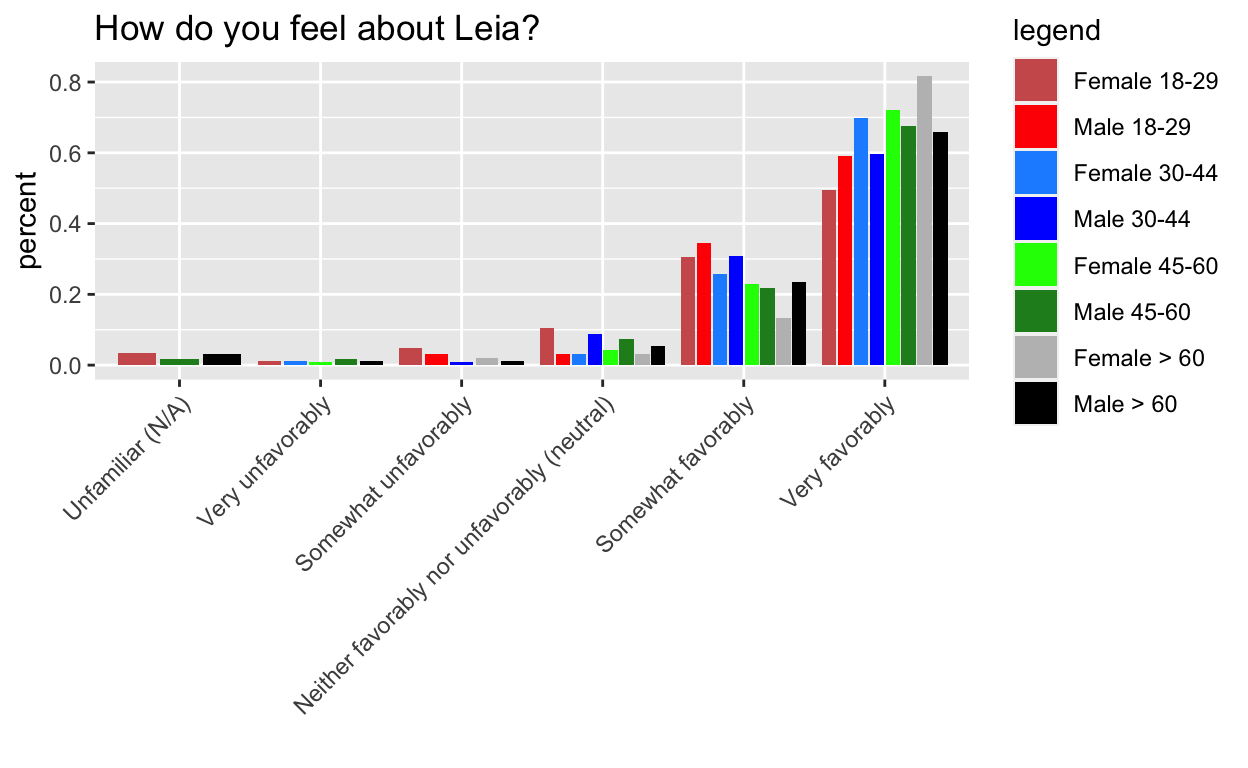``````## make colors more informative (light and dark of a color for male and female same age), rearrange levels so easier to compare
``````

#### Challenges

1. Side by side instead of stacked (`geom_bar` documentation)

2. Change barchart colors.

3. Rotate axis labels.

4. Releveling. In haste, I did not do this well. Read this to see why I’m wrong and how I could do better.

5. Normalizing by the number per category. Letting `stat="count"` was a red herring. It would be nice if there was a way to input values to normalize the fill variable by, but instead I ended up manually calculating the percentages and using `stat="identity"`.

6. Correct and consistent ordering of colors (to match the legend) Thanks for the pointers @ibddoctor and @hadleywickham!